# Use a normal approximation to find the probability of the indicated number of voters. In this...

Use a normal approximation to find the probability of the indicated number of voters. In this case, assume that 156 eligible voters aged 18-24 are randomly selected. Suppose a previous study showed that among eligible voters aged 18-24,22 % of them voted.

Probability that fewer than 38 voted

The probability that fewer than 38 of 156 eligible voters voted is _______

(Round to four decimal places as needed.)

Solution

\begin{aligned} &\text { given : } n=156, p(\text { eligible voters })=0.22 \\ &n p=156 * 0.22=34.32 \geq 5 \\ &n(1-p)=156 *(1-0.22)=121.68 \geq 5 \end{aligned}

$$\therefore$$ Binomial random variable is approximately normal

$$\operatorname{Mean}(\mu)=n p=156 * 0.22=34.32$$

Standard deviation $$(\sigma)=\sqrt{n p(1-p)}=\sqrt{156 * 0.22 *(1-0.22)}=\sqrt{26.7696}$$ formula : $$Z=\frac{X-\mu}{\sigma}$$

$$P($$ fewer than 38) \Rightarrow P(X Use continuity correction.  \begin{aligned} &P(X<a)=P(X<a-0.5) \\ &\Rightarrow P(X<37.5) \\ &\Rightarrow P\left(\frac{X-\mu}{\sigma}<\frac{37.5-34.32}{\sqrt{26.7696}}\right) \\ &\Rightarrow P(Z<0.61) \end{aligned}  Refer to Z-table to find the probability or use the excel formula "=NORM.S.DIST(0.61, TRUE)" to find the probability.  \Rightarrow 0.7306  \(\therefore The probability that fewer than 38 of 156 eligible voters voted is $$0.7306$$

#### Earn Coin

Coins can be redeemed for fabulous gifts.

Similar Homework Help Questions
• ### Use a normal approximation to find the probability of the indicated number of voters. In this...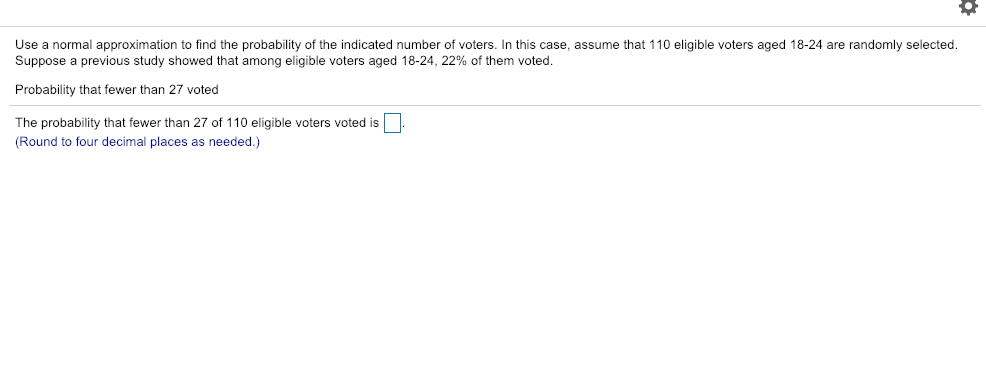Use a normal approximation to find the probability of the indicated number of voters. In this case, assume that 110 eligible voters aged 18-24 are randomly selected Suppose a previous study showed that among eligible voters aged 18-24, 22% of them voted. Probability that fewer than 27 voted The probability that fewer than 27 of 110 eligible voters voted is Round to four decimal places as needed.)

• ### Use a normal approximation to find the probability of the indicated number of voters. In this...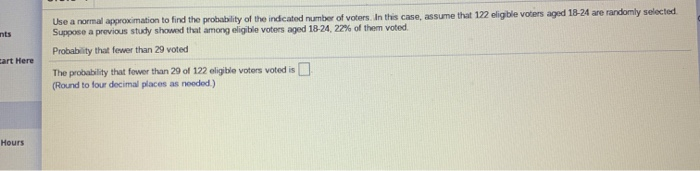Use a normal approximation to find the probability of the indicated number of voters. In this case, assume that 122 eligible voters aged 18-24 are randomly selected Suppose a previous study showed that among eligible voters aged 18-24, 22% of them voted Probability that fewer than 29 voted The probability that fewer than 29 of 122 eligible voters voted is (Round to four decimal places as needed.) Hours

• ### Use a normal approximation to find the probability of the indicated number of voters. In this​...

Use a normal approximation to find the probability of the indicated number of voters. In this​ case, assume that 198 198 eligible voters aged​ 18-24 are randomly selected. Suppose a previous study showed that among eligible voters aged​ 18-24, 22% of them voted. Probability that fewer than 49 49 voted The probability that fewer than 49 49 of 198 198 eligible voters voted is nothing . ​(Round to four decimal places as​ needed.)

• ### Use a normal approximation to find the probability of the indicated number of voters. In this​...

Use a normal approximation to find the probability of the indicated number of voters. In this​ case, assume that107 eligible voters aged​ 18-24 are randomly selected. Suppose a previous study showed that among eligible voters aged 18-24, 22% of them voted. Probability that fewer than 28 voted The probability that fewer than 28 of 107 eligible voters voted is_______

• ### Use a normal approximation to find the probability of the indicated number of voters. In this...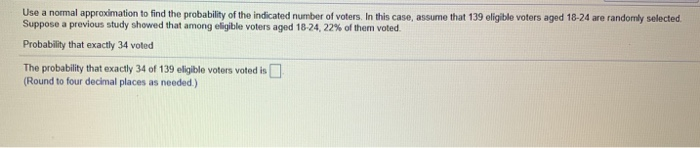Use a normal approximation to find the probability of the indicated number of voters. In this case, assume that 139 eligible voters aged 18-24 are randomly selected Suppose a previous study showed that among eligible voters aged 18-24, 22% of them voted Probability that exactly 34 voted The probability that exactly 34 of 139 eligible voters voted is (Round to four decimal places as needed.)

• ### Use a normal approximation to find the probability of the indicated number of voters. In this...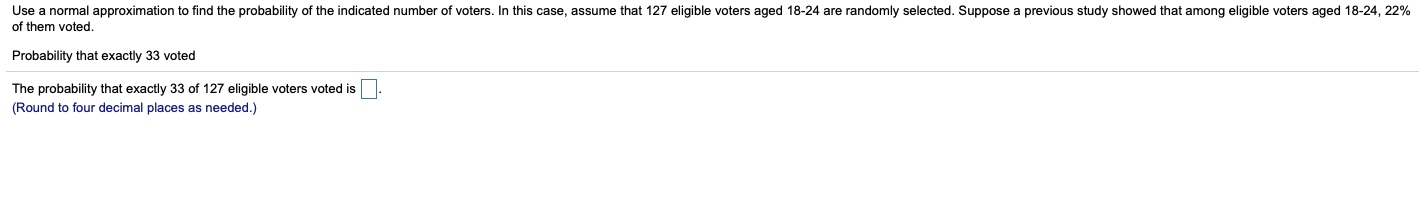Use a normal approximation to find the probability of the indicated number of voters. In this case, assume that 127 eligible voters aged 18-24 are randomly selected. Suppose a previous study showed that among eligible voters aged 18-24, 22% of them voted. Probability that exactly 33 voted The probability that exactly 33 of 127 eligible voters voted is (Round to four decimal places as needed.)

• ### Use a normal approximation to find the probability of the indicated number of voters. In this...

Use a normal approximation to find the probability of the indicated number of voters. In this case, assume that 182 eligible voters aged 18-24 are randomly selected. Suppose a previous study showed that among eligible voters aged 18-24,22% of them voted.Probability that exactly 43 voted.The probability that exactly 43 of 182 eligible voters voted is _______

• ### This Question: 1 pt 4 of 35 (10 complete) This Test: 35 pts possible Use a...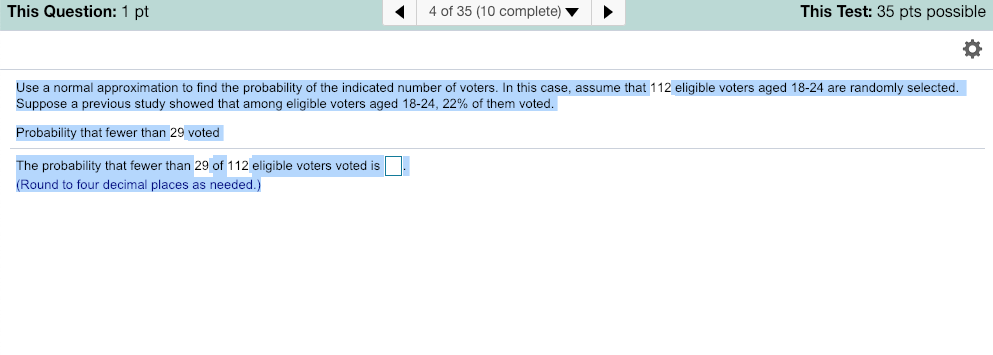This Question: 1 pt 4 of 35 (10 complete) This Test: 35 pts possible Use a normal approximation to find the probability of the indicated number of voters. In this case, assume that 112 eligible voters aged 18-24 are randomly selected Suppose a previous study showed that among eligible voters aged 18-24, 22% of them voted. Probability that fewer than 29 voted The probability that fewer than 29 of 112 eligible voters voted is (Round to four decimal places as...

• ### Question Help Use a normal approximation to find the probability of the indicated number of voters....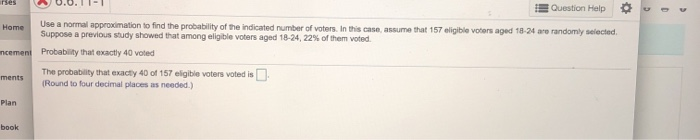Question Help Use a normal approximation to find the probability of the indicated number of voters. In this case, assume that 157 suppose a previous study showed that among eligible voters aged 18-24, 22% of them voted eligible votors aged 18-24 are randomly selected. ncement Probability that exactly 40 voted ments The probeblity that exacty 40 of 157 eligible voters voted is ets(Round to four decimal places as needed.) probability that exacty 40 of 157 eligible voters voted is Plan...

• ### If np 25 and nq 25, estimate P(more than 9) with n = 12 and p...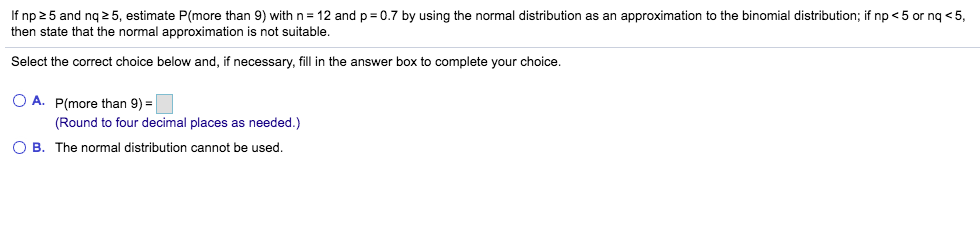If np 25 and nq 25, estimate P(more than 9) with n = 12 and p = 0.7 by using the normal distribution as an approximation to the binomial distribution, if np < 5 or nq <5, then state that the normal approximation is not suitable. Select the correct choice below and, if necessary, fill in the answer box to complete your choice. O A. P(more than 9) = (Round to four decimal places as needed.) OB. The normal distribution...